MathematicsExamples: Applying the ODE Initial Value Problem Solvers

This section contains several examples that illustrate the kinds of problems you can solve. For each example, there is a corresponding M-file, included in MATLAB. You can

• View the M-file code in an editor by entering edit followed by the name of the M-file at the MATLAB prompt. For example, to view the code for the simple nonstiff problem example, enter
• edit rigidode

• Alternatively, if you are reading this in the MATLAB Help Browser, you can click the name of the M-file in the list below.

• Run the example by entering the name of the M-file at the MATLAB prompt.

This section presents the following examples:

Example: Simple Nonstiff Problem

rigidode illustrates the solution of a standard test problem proposed by Krogh for solvers intended for nonstiff problems .

The ODEs are the Euler equations of a rigid body without external forces.

•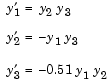For your convenience, the entire problem is defined and solved in a single M-file. The differential equations are coded as a subfunction f. Because the example calls the ode45 solver without output arguments, the solver uses the default output function odeplot to plot the solution components.

To run this example, click on the example name, or type rigidode at the command line.

• function rigidode
%RIGIDODE  Euler equations of a rigid body without external forces
tspan = [0 12];
y0 = [0; 1; 1];

% Solve the problem using ode45
ode45(@f,tspan,y0);
% ------------------------------------------------------------
function dydt = f(t,y)
dydt = [ y(2)*y(3)
-y(1)*y(3)
-0.51*y(1)*y(2) ];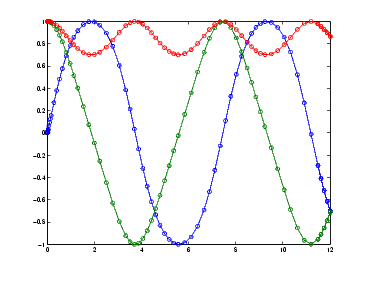Example: Stiff Problem (van der Pol Equation)

vdpode illustrates the solution of the van der Pol problem described in Example: The van der Pol Equation, µ = 1000 (Stiff). The differential equations

•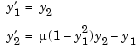involve a constant parameter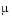.

Asincreases, the problem becomes more stiff, and the period of oscillation becomes larger. Whenis 1000 the equation is in relaxation oscillation and the problem is very stiff. The limit cycle has portions where the solution components change slowly and the problem is quite stiff, alternating with regions of very sharp change where it is not stiff (quasi-discontinuities).

By default, the solvers in the ODE suite that are intended for stiff problems approximate Jacobian matrices numerically. However, this example provides a nested function J(t,y) to evaluate the Jacobian matrix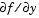analytically at (t,y) for= MU. The use of an analytic Jacobian can improve the reliability and efficiency of integration.

To run this example, click on the example name, or type vdpode at the command line. From the command line, you can specify a value ofas an argument to vdpode. The default is= 1000.

• function vdpode(MU)
%VDPODE  Parameterizable van der Pol equation (stiff for large MU)
if nargin < 1
MU = 1000;     % default
end

tspan = [0; max(20,3*MU)];              % Several periods
y0 = [2; 0];
options = odeset('Jacobian',@J);

[t,y] = ode15s(@f,tspan,y0,options);

plot(t,y(:,1));
title(['Solution of van der Pol Equation, \mu = ' num2str(MU)]);
xlabel('time t');
ylabel('solution y_1');

axis([tspan(1) tspan(end) -2.5 2.5]);
---------------------------------------------------------------
function dydt = f(t,y)
dydt = [            y(2)
MU*(1-y(1)^2)*y(2)-y(1) ];
end   % End nested function f
---------------------------------------------------------------
function dfdy = J(t,y)
dfdy = [         0                  1
-2*MU*y(1)*y(2)-1    MU*(1-y(1)^2) ];
end   % End nested function J
end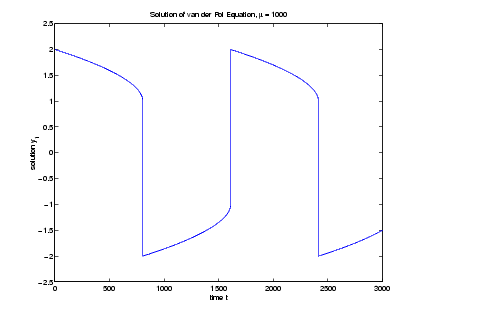Example: Finite Element Discretization

fem1ode illustrates the solution of ODEs that result from a finite element discretization of a partial differential equation. The value of N in the call fem1ode(N) controls the discretization, and the resulting system consists of N equations. By default, N is 19.

This example involves a mass matrix. The system of ODEs comes from a method of lines solution of the partial differential equation

•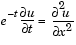with initial condition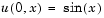and boundary conditions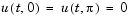. An integer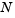is chosen,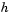is defined as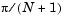, and the solution of the partial differential equation is approximated at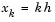for k = 0, 1, ..., N+1 by

•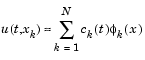Here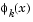is a piecewise linear function that is 1 at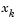and 0 at all the other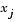. A Galerkin discretization leads to the system of ODEs

•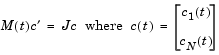and the tridiagonal matrices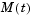and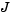are given by

•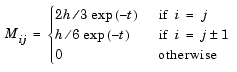and

•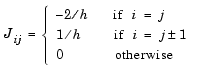The initial values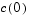are taken from the initial condition for the partial differential equation. The problem is solved on the time interval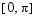.

In the fem1ode example, the properties

• options = odeset('Mass',@mass,'MStateDep','none','Jacobian',J)


indicate that the problem is of the form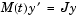. The nested function mass(t) evaluates the time-dependent mass matrixand J is the constant Jacobian.

To run this example, click on the example name, or type fem1ode at the command line. From the command line, you can specify a value ofas an argument to fem1ode. The default is= 19.

• function fem1ode(N)
%FEM1ODE  Stiff problem with a time-dependent mass matrix

if nargin < 1
N = 19;
end
h = pi/(N+1);
y0 = sin(h*(1:N)');
tspan = [0; pi];

% The Jacobian is constant.
e = repmat(1/h,N,1);    %  e=[(1/h) ... (1/h)];
d = repmat(-2/h,N,1);   %  d=[(-2/h) ... (-2/h)];
J = spdiags([e d e], -1:1, N, N);

options = odeset('Mass',@mass,'MStateDependence','none', ...
'Jacobian',J);

[t,y] = ode15s(@f,tspan,y0,options);

surf((1:N)/(N+1),t,y);
set(gca,'ZLim',[0 1]);
view(142.5,30);
title(['Finite element problem with time-dependent mass ' ...
'matrix, solved by ODE15S']);
xlabel('space ( x/\pi )');
ylabel('time');
zlabel('solution');
%--------------------------------------------------------------
-
function out = f(t,y)
h = pi/(N+1);
e = repmat(1/h,N,1);    %  e=[(1/h) ... (1/h)];
d = repmat(-2/h,N,1);   %  d=[(-2/h) ... (-2/h)];
J = spdiags([e d e], -1:1, N, N);
out = J*y;
end   % End nested function f
%--------------------------------------------------------------
-
function M = mass(t)
h = pi/(N+1);
e = repmat(exp(-t)*h/6,N,1);  % e(i)=exp(-t)*h/6
e4 = repmat(4*exp(-t)*h/6,N,1);
M = spdiags([e e4 e], -1:1, N, N);
end   % End nested function mass
end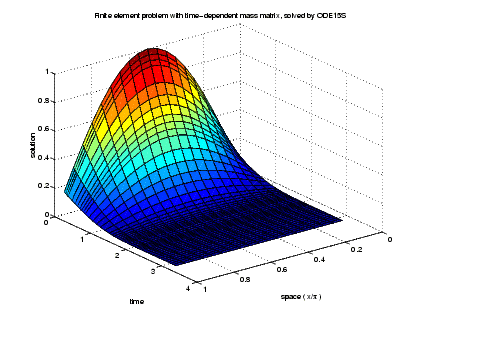Example: Large, Stiff, Sparse Problem

brussode illustrates the solution of a (potentially) large stiff sparse problem. The problem is the classic "Brusselator" system  that models diffusion in a chemical reaction

•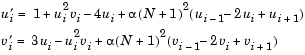and is solved on the time interval [0,10] with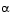= 1/50 and

•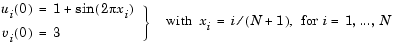There are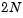equations in the system, but the Jacobian is banded with a constant width 5 if the equations are ordered as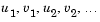In the call brussode(N), where N corresponds to, the parameter N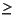2 specifies the number of grid points. The resulting system consists of 2N equations. By default, N is 20. The problem becomes increasingly stiff and the Jacobian increasingly sparse as N increases.

The nested function f(t,y) returns the derivatives vector for the Brusselator problem. The subfunction jpattern(N) returns a sparse matrix of 1s and 0s showing the locations of nonzeros in the Jacobian. The example assigns this matrix to the property JPattern, and the solver uses the sparsity pattern to generate the Jacobian numerically as a sparse matrix. Providing a sparsity pattern can significantly reduce the number of function evaluations required to generate the Jacobian and can accelerate integration.

For the Brusselator problem, if the sparsity pattern is not supplied, 2N evaluations of the function are needed to compute the 2N-by-2N Jacobian matrix. If the sparsity pattern is supplied, only four evaluations are needed, regardless of the value of N.

To run this example, click on the example name, or type brussode at the command line. From the command line, you can specify a value ofas an argument to brussode. The default is= 20.

• function brussode(N)
%BRUSSODE  Stiff problem modeling a chemical reaction

if nargin < 1
N = 20;
end

tspan = [0; 10];
y0 = [1+sin((2*pi/(N+1))*(1:N));
repmat(3,1,N)];

options = odeset('Vectorized','on','JPattern',jpattern(N));

[t,y] = ode15s(@f,tspan,y0,options);

u = y(:,1:2:end);
x = (1:N)/(N+1);
surf(x,t,u);
view(-40,30);
xlabel('space');
ylabel('time');
zlabel('solution u');
title(['The Brusselator for N = ' num2str(N)]);
% --------------------------------------------------------------
function dydt = f(t,y)
c = 0.02 * (N+1)^2;
dydt = zeros(2*N,size(y,2));      % preallocate dy/dt
% Evaluate the two components of the function at one edge of
% the grid (with edge conditions).
i = 1;
dydt(i,:) = 1 + y(i+1,:).*y(i,:).^2 - 4*y(i,:) + ...
c*(1-2*y(i,:)+y(i+2,:));
dydt(i+1,:) = 3*y(i,:) - y(i+1,:).*y(i,:).^2 + ...
c*(3-2*y(i+1,:)+y(i+3,:));
% Evaluate the two components of the function at all interior
% grid points.
i = 3:2:2*N-3;
dydt(i,:) = 1 + y(i+1,:).*y(i,:).^2 - 4*y(i,:) + ...
c*(y(i-2,:)-2*y(i,:)+y(i+2,:));
dydt(i+1,:) = 3*y(i,:) - y(i+1,:).*y(i,:).^2 + ...
c*(y(i-1,:)-2*y(i+1,:)+y(i+3,:));
% Evaluate the two components of the function at the other edge
% of the grid (with edge conditions).
i = 2*N-1;
dydt(i,:) = 1 + y(i+1,:).*y(i,:).^2 - 4*y(i,:) + ...
c*(y(i-2,:)-2*y(i,:)+1);
dydt(i+1,:) = 3*y(i,:) - y(i+1,:).*y(i,:).^2 + ...
c*(y(i-1,:)-2*y(i+1,:)+3);
end   % End nested function f
end   % End function brussode
% --------------------------------------------------------------
function S = jpattern(N)
B = ones(2*N,5);
B(2:2:2*N,2) = zeros(N,1);
B(1:2:2*N-1,4) = zeros(N,1);
S = spdiags(B,-2:2,2*N,2*N);
end;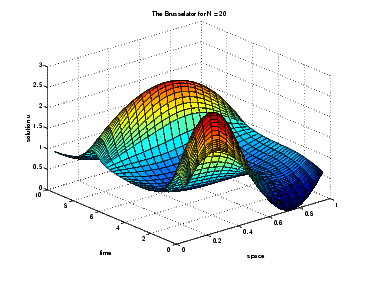Example: Simple Event Location

ballode models the motion of a bouncing ball. This example illustrates the event location capabilities of the ODE solvers.

The equations for the bouncing ball are

•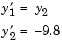In this example, the event function is coded in a subfunction events

• [value,isterminal,direction] = events(t,y)


which returns

• A value of the event function
• The information whether or not the integration should stop when value = 0 (isterminal = 1 or 0, respectively)
• The desired directionality of the zero crossings:

 -1 Detect zero crossings in the negative direction only 0 Detect all zero crossings 1 Detect zero crossings in the positive direction only

The length of value, isterminal, and direction is the same as the number of event functions. The ith element of each vector, corresponds to the ith event function. For an example of more advanced event location, see orbitode (Example: Advanced Event Location).

In ballode, setting the Events property to @events causes the solver to stop the integration (isterminal = 1) when the ball hits the ground (the height y(1) is 0) during its fall (direction = -1). The example then restarts the integration with initial conditions corresponding to a ball that bounced.

To run this example, click on the example name, or type ballode at the command line.

• function ballode
%BALLODE  Run a demo of a bouncing ball.

tstart = 0;
tfinal = 30;
y0 = [0; 20];
refine = 4;
options = odeset('Events',@events,'OutputFcn', @odeplot,...
'OutputSel',1,'Refine',refine);

set(gca,'xlim',[0 30],'ylim',[0 25]);
box on
hold on;

tout = tstart;
yout = y0.';
teout = [];
yeout = [];
ieout = [];
for i = 1:10
% Solve until the first terminal event.
[t,y,te,ye,ie] = ode23(@f,[tstart tfinal],y0,options);
if ~ishold
hold on
end
% Accumulate output.
nt = length(t);
tout = [tout; t(2:nt)];
yout = [yout; y(2:nt,:)];
teout = [teout; te];    % Events at tstart are never reported.
yeout = [yeout; ye];
ieout = [ieout; ie];

ud = get(gcf,'UserData');
if ud.stop
break;
end

% Set the new initial conditions, with .9 attenuation.
y0(1) = 0;
y0(2) = -.9*y(nt,2);

% A good guess of a valid first time step is the length of
% the last valid time step, so use it for faster computation.
options = odeset(options,'InitialStep',t(nt)-t(nt-refine),...
'MaxStep',t(nt)-t(1));
tstart = t(nt);
end

plot(teout,yeout(:,1),'ro')
xlabel('time');
ylabel('height');
title('Ball trajectory and the events');
hold off
odeplot([],[],'done');
% --------------------------------------------------------------
function dydt = f(t,y)
dydt = [y(2); -9.8];
% --------------------------------------------------------------
function [value,isterminal,direction] = events(t,y)
% Locate the time when height passes through zero in a
% decreasing direction and stop integration.
value = y(1);     % Detect height = 0
isterminal = 1;   % Stop the integration
direction = -1;   % Negative direction only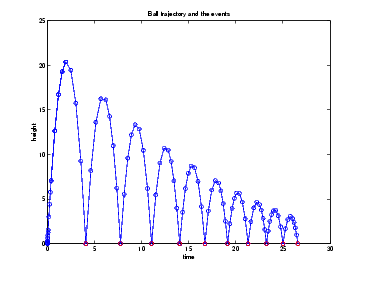Example: Advanced Event Location

orbitode illustrates the solution of a standard test problem for those solvers that are intended for nonstiff problems. It traces the path of a spaceship traveling around the moon and returning to the earth. (Shampine and Gordon , p.246).

The orbitode problem is a system of the following four equations shown:

•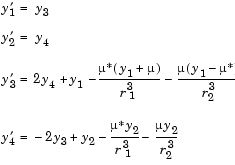where

•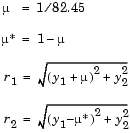The first two solution components are coordinates of the body of infinitesimal mass, so plotting one against the other gives the orbit of the body. The initial conditions have been chosen to make the orbit periodic. The value ofcorresponds to a spaceship traveling around the moon and the earth. Moderately stringent tolerances are necessary to reproduce the qualitative behavior of the orbit. Suitable values are 1e-5 for RelTol and 1e-4 for AbsTol.

The nested events function includes event functions that locate the point of maximum distance from the starting point and the time the spaceship returns to the starting point. Note that the events are located accurately, even though the step sizes used by the integrator are not determined by the location of the events. In this example, the ability to specify the direction of the zero crossing is critical. Both the point of return to the initial point and the point of maximum distance have the same event function value, and the direction of the crossing is used to distinguish them.

To run this example, click on the example name, or type orbitode at the command line. The example uses the output function odephas2 to produce the two-dimensional phase plane plot and let you to see the progress of the integration.

• function orbitode
%ORBITODE  Restricted three-body problem

mu = 1 / 82.45;
mustar = 1 - mu;
y0 = [1.2; 0; 0; -1.04935750983031990726];
tspan = [0 7];

options = odeset('RelTol',1e-5,'AbsTol',1e-4,...
'OutputFcn',@odephas2,'Events',@events);

[t,y,te,ye,ie] = ode45(@f,tspan,y0,options);

plot(y(:,1),y(:,2),ye(:,1),ye(:,2),'o');
title ('Restricted three body problem')
ylabel ('y(t)')
xlabel ('x(t)')
% --------------------------------------------------------------
function dydt = f(t,y)
r13 = ((y(1) + mu)^2 + y(2)^2) ^ 1.5;
r23 = ((y(1) - mustar)^2 + y(2)^2) ^ 1.5;
dydt = [ y(3)
y(4)
2*y(4) + y(1) - mustar*((y(1)+mu)/r13) - ...
mu*((y(1)-mustar)/r23)
-2*y(3) + y(2) - mustar*(y(2)/r13) - mu*(y(2)/r23) ];
end   % End nested function f
% --------------------------------------------------------------
function [value,isterminal,direction] = events(t,y)
% Locate the time when the object returns closest to the
% initial point y0 and starts to move away, and stop integration.
% Also locate the time when the object is farthest from the
% initial point y0 and starts to move closer.
%
% The current distance of the body is
%
%   DSQ = (y(1)-y0(1))^2 + (y(2)-y0(2))^2
%       = <y(1:2)-y0(1:2),y(1:2)-y0(1:2)>
%
% A local minimum of DSQ occurs when d/dt DSQ crosses zero
% heading in the positive direction.  We can compute d(DSQ)/dt as
%
%  d(DSQ)/dt = 2*(y(1:2)-y0(1:2))'*dy(1:2)/dt = ...
%                 2*(y(1:2)-y0(1:2))'*y(3:4)
%
dDSQdt = 2 * ((y(1:2)-y0(1:2))' * y(3:4));
value = [dDSQdt; dDSQdt];
isterminal = [1; 0];            % Stop at local minimum
direction = [1; -1];            % [local minimum, local maximum]
end   % End nested function events
end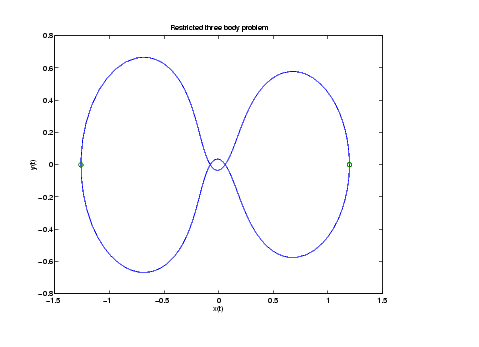Example: Differential-Algebraic Problem

hb1dae reformulates the hb1ode example as a differential-algebraic equation (DAE) problem. The Robertson problem coded in hb1ode is a classic test problem for codes that solve stiff ODEs.

•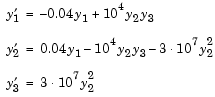Note    The Robertson problem appears as an example in the prolog to LSODI .

In hb1ode, the problem is solved with initial conditions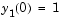,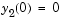,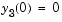to steady state. These differential equations satisfy a linear conservation law that is used to reformulate the problem as the DAE

•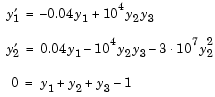Obviously these equations do not have a solution for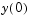with components that do not sum to 1. The problem has the form of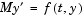with

•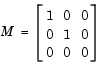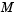is obviously singular, but hb1dae does not inform the solver of this. The solver must recognize that the problem is a DAE, not an ODE. Similarly, although consistent initial conditions are obvious, the example uses an inconsistent value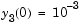to illustrate computation of consistent initial conditions.

To run this example, click on the example name, or type hb1dae at the command line. Note that hb1dae:

• Imposes a much smaller absolute error tolerance on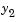than on the other components. This is becauseis much smaller than the other components and its major change takes place in a relatively short time.
• Specifies additional points at which the solution is computed to more clearly show the behavior of.
• Multipliesby 104 to makevisible when plotting it with the rest of the solution.
• Uses a logarithmic scale to plot the solution on the long time interval.
• function hb1dae
%HB1DAE  Stiff differential-algebraic equation (DAE)

% A constant, singular mass matrix
M = [1 0 0
0 1 0
0 0 0];

% Use an inconsistent initial condition to test initialization.
y0 = [1; 0; 1e-3];
tspan = [0 4*logspace(-6,6)];

% Use the LSODI example tolerances. The 'MassSingular' property
% is left at its default 'maybe' to test the automatic detection
% of a DAE.
options = odeset('Mass',M,'RelTol',1e-4,...
'AbsTol',[1e-6 1e-10 1e-6],'Vectorized','on');

[t,y] = ode15s(@f,tspan,y0,options);

y(:,2) = 1e4*y(:,2);

semilogx(t,y);
ylabel('1e4 * y(:,2)');
title(['Robertson DAE problem with a Conservation Law, '...
'solved by ODE15S']);
xlabel('This is equivalent to the stiff ODEs coded in HB1ODE.');
% --------------------------------------------------------------
function out = f(t,y)
out = [ -0.04*y(1,:) + 1e4*y(2,:).*y(3,:)
0.04*y(1,:) - 1e4*y(2,:).*y(3,:) - 3e7*y(2,:).^2
y(1,:) + y(2,:) + y(3,:) - 1 ];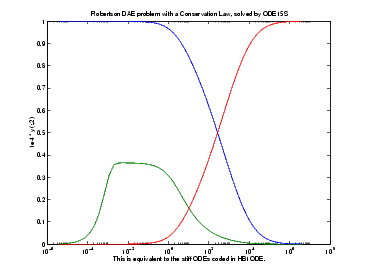Summary of Code Examples

The following table lists the M-files for all the ODE initial value problem examples. Click the example name to see the code in an editor. Type the example name at the command line to run it.

 Note    The Differential Equations Examples browser enables you to view the code for the ODE examples and DAE examples. You can also run the examples from the browser. Click on these links to invoke the browser, or type odeexamples('ode')or odeexamples('dae')at the command line.

 Example Description amp1dae Stiff DAE -- electrical circuit ballode Simple event location -- bouncing ball batonode ODE with time- and state-dependent mass matrix -- motion of a baton brussode Stiff large problem -- diffusion in a chemical reaction (the Brusselator) burgersode ODE with strongly state-dependent mass matrix -- Burger's equation solved using a moving mesh technique fem1ode Stiff problem with a time-dependent mass matrix -- finite element method fem2ode Stiff problem with a constant mass matrix -- finite element method hb1ode Stiff ODE problem solved on a very long interval -- Robertson chemical reaction hb1dae Robertson problem -- stiff, linearly implicit DAE from a conservation law ihb1dae Robertson problem -- stiff, fully implicit DAE iburgersode Burgers' equation solved as implicit ODE system orbitode Advanced event location -- restricted three body problem rigidode Nonstiff problem -- Euler equations of a rigid body without external forces vdpode Parameterizable van der Pol equation (stiff for large)Changing ODE Integration Properties Questions and Answers, and Troubleshooting© 1994-2005 The MathWorks, Inc.